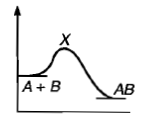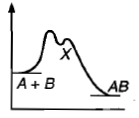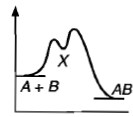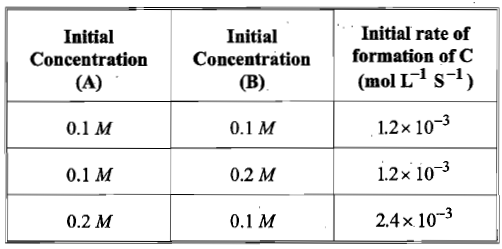For a certain reaction a plot of [(c0-c)/c] against time t, yields a straight line. c0 and c are concentrations of reactant at t = 0 and t = t respectively. The order of reaction is:

(a) 3

(b) 0

(c) 1

(d) 2

High Yielding Test Series + Question Bank - NEET 2020

Difficulty Level:

Hydrogenation of vegetable ghee at 250C reduces pressure of H2 from 2 atom to 1.2 atom in 50 minute. The rate of reaction in terms of molarity per second is:

(a)1.09 x 10-6

(b)1.09 x 10-5

(c)1.09 x 10-7

(d)1.09 x 10-8

High Yielding Test Series + Question Bank - NEET 2020

Difficulty Level:

A hypothetical reaction, A2 +B2 $\to$ 2AB mechanism as given below;

A2 $⇌$A+ A          ............(Fast)

A+B2 → AB + B   ............(Slow)

A+ → AB         ............(Fast)

The order of the overall reaction is:

1. 2

2. 1

3. 1.5

4. zero

High Yielding Test Series + Question Bank - NEET 2020

Difficulty Level:

Following mechanism has been proposed for a reaction,

2A+B $\to$D+E

A+B$\to$ C+D    ...(Slow)

A+ C$\to$ E         ...(Fast)

The rate law expression for the reaction is:

(a) r = K[A]2[B]

(b) r=K[A][B]

(c) r= K[A]2

(d) r= K[A][C]

High Yielding Test Series + Question Bank - NEET 2020

Difficulty Level:

In gaseous reactions important for the understanding of the upper atmosphere H2O and O react bimolecularly to form two OH radicals. $∆$H for this reaction is 72kJ at 500 K and Ea is 77 kJ mol-1, then Ea for the bimolecular recombination of two OH radicals to form H2O and O is:

(a) 3 kJ mol-1

(b) 4 kJ mol-1

(c) 5 kJ mol-1

(d) 7 kJ mol-1

High Yielding Test Series + Question Bank - NEET 2020

Difficulty Level:

For a reaction A Product, rate law is $-\frac{d\left[A\right]}{dt}=K\left[A{\right]}_{0}$. The concentration of A left after time t when $t=\frac{1}{K}$ is:

(a) $\frac{\left[A{\right]}_{0}}{e}$

(b)

(c) $\frac{\left[A{\right]}_{0}}{{e}^{2}}$

(d) $\frac{1}{\left[A{\right]}_{0}}$

High Yielding Test Series + Question Bank - NEET 2020

Difficulty Level:

For an exothermic chemical process occurring in two steps as;

(i) A+B$\to$X(Slow)

(ii) X$\to$AB (Fast)

The progress of the reaction can be best described by:

(a)(b)(c)(d) all of these

High Yielding Test Series + Question Bank - NEET 2020

Difficulty Level:

For the non-stoichiometric reaction 2A + B $\to$ C +D, the following kinetic data were obtained in three separate experiments, all at 298 K.The rate law for the formation of C is:

(a) d[C]/dt = k[ A][B]

(b) d[C]/dt = k[ A]2[B]

(c) d[C]/dt = k[ A][B]2

(d) d[C]/dt = k[ A]

High Yielding Test Series + Question Bank - NEET 2020

Difficulty Level:

For the reaction N2 + 3H2 $\to$ 2NH3, the rate d[NH3]/dt = 2x10-4 Ms-1 .Therefore, the rate -d[N2]/dt is given as:

(a) 10-4 Ms-1

(b) 104 Ms-1

(c) 10-2 sM-1

(d) 10-4 sM-1

High Yielding Test Series + Question Bank - NEET 2020

Difficulty Level:

If 'I' is the intensity of absorbed light and 'c' is the concentration of AB for the photochemical process AB + hv→  AB *, the rate of formation of AB * is directly proportional to:

(a) c

(b) I

(c) I2

(d) cI